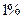Chapter 33, Problem 35P### Principles of Economics 2e

2nd Edition
Steven A. Greenlaw; David Shapiro
ISBN: 9781947172364

#### Solutions

Chapter
Section### Principles of Economics 2e

2nd Edition
Steven A. Greenlaw; David Shapiro
ISBN: 9781947172364
Textbook Problem

# If trade increases world GDP byper year, what is the global impact of this increase over 10 years? How does this increase compare to the annual GDP of a country like Sri Lanka? Discuss. Hint To answer this question, here are steps you may want to consider. Go to the World Development Indicators (online) published by the World Bank. Find the current level of World GDP in constant international dollars. Also, find the GDP of Sri Lanka in constant international dollars. Once you have these two numbers, compute the amount the additional increase in global incomes due to trade and compare that number to Sri Lanka’s GDP.

To determine

The global impact of increase in world GDP by 1% per year due to increase in trade over 10 years is to be determined along with the comparison of this increase to the annual GDP of country like Sri Lanka.

Explanation

Now, as given in the question that on the increase in the trade the GDP of the world will increase by 1% per year which gives the following value over the ten years:

Global GDP=(1+0.01)10=1.105 times

It means the impact will be that the GDP will be 1.105 times the GDP of the world which is at the starting of the ten years.

For example, the GDP of world at year 1 is $1000 millions then the GDP at the end of year 10 will be: GDP at Year 10=$1000 millions×(1+0.01)10=$1000 millions×1.105=$1105 millions

Now, the current level of world GDP in constant international dollars is \$82,968 billion

### Still sussing out bartleby?

Check out a sample textbook solution.

See a sample solution

#### The Solution to Your Study Problems

Bartleby provides explanations to thousands of textbook problems written by our experts, many with advanced degrees!

Get Started

#### Find more solutions based on key concepts